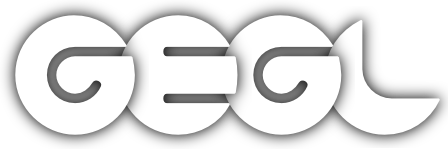## GEGL graph

Do a chain of operations, with key=value pairs after each operation name to set properties. And aux=[ source filter ] for specifying a chain with a source as something connected to an aux pad.
pipeline
[op [property=value] [property=value]] [[op] [property=value]
name: string type: string default: # uncomment a set of lines below by removing the\n# leading to test and modify an example, use\n# use ctrl+a before typing to select all, if you\n# want a blank slate.\n#\nid=in # name a reference to the input buffer 'in'\n\n\n# adaptive threshold:\n#\n#threshold aux=[ ref=in gaussian-blur std-dev-x=0.2rel std-dev-y=0.2rel ]\n\n# local white balance and contrast stretching\n#\n#divide aux=[ ref=in median-blur radius=.25rel percentile=100 gaussian-blur std-dev-x=.5rel std-dev-y=.5rel ]\n\n# median sharpen (unsharp-mask with median-blur):\n#\n#add aux=[ ref=in subtract aux=[ ref=in median-blur radius=5 ] ] \n\n# styled text overlay\n#\n#over aux=[ text wrap=1.0rel color=rgb(0.1,0.1,.3) size=.1rel string=\"ipsum sic amet deliriarium mic sel adendum. Mic fubar bax qux facilium dhat.\" dropshadow radius=.01rel grow-radius=0.0065rel color=white x=0 y=0 border-align x=0.5 y=0.33 ] # try x=1 y=1\n\n\n# thumbs with misc filters along bottom of image:\n#\n#over aux=[\n# ref=in scale-ratio x=0.20 y=0.20 newsprint period=0.01rel period2=0.01rel period3=0.01rel period4=0.01rel color-model=cmyk aa-samples=64 pattern=pssquare pattern2=pssquare pattern3=pssquare pattern4=pssquare \n# pack gap=0.05rel aux=[ ref=in scale-ratio x=0.20 y=0.20 newsprint period=0.01rel period2=0.01rel period3=0.01rel period4=00.01rel color-model=rgb aa-samples=64 ] \n# pack gap=0.05rel aux=[ ref=in scale-ratio x=0.20 y=0.20 id=scaled snn-mean snn-mean crop aux=[ ref=scaled ] ] \n# pack gap=0.05rel aux=[ ref=in scale-ratio x=0.20 y=0.20 mosaic tile-size=0.03rel ] \n# border-align x=0.5 y=0.9\n#]\n\n# All the examples can be expanded to be on\n# multiple lines, this graph description\n# language is not whitespace sensitive, the\n# rel suffix is relative to image height\n multiline:true
Eeeeeek
There is a problem in the syntax or in the application of parsed property values. Things might mostly work nevertheless.
name: error type: string default:  error:true
name: gegl:geglThis page is part of the online GEGL Documentation, GEGL is a data flow based image processing library/framework, made to fuel GIMPs high-bit depth non-destructive editing future.